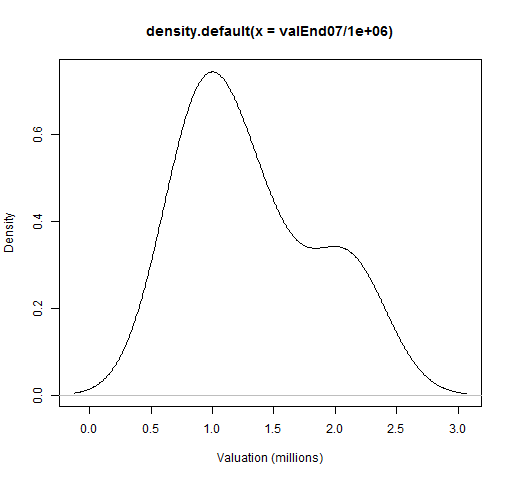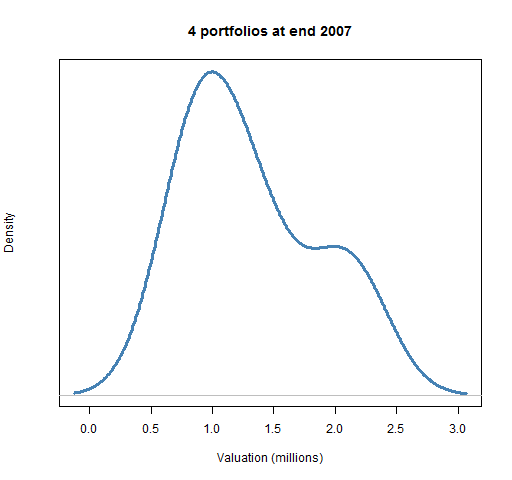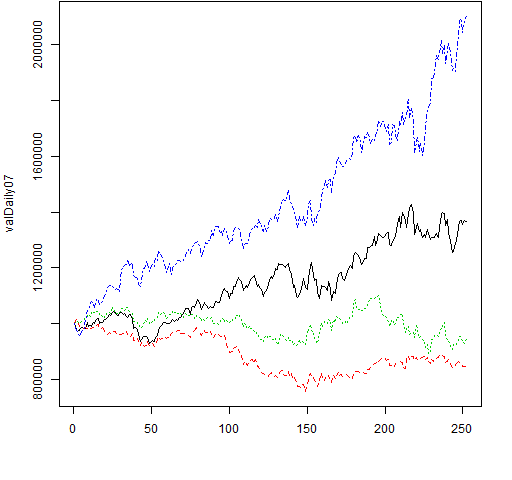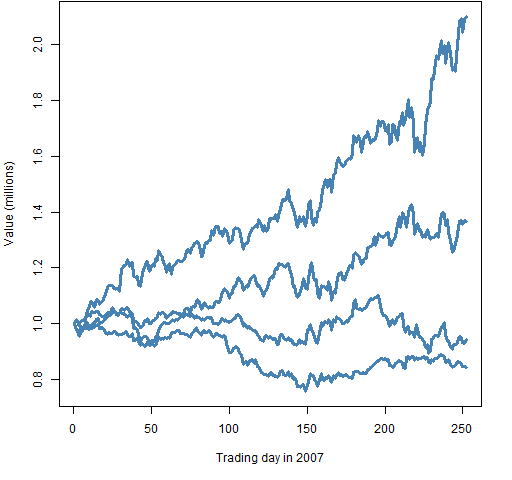## Create and plot valuations

Compute the valuation of a set of random portfolios at one or more times.  These can then be plotted.

## Preparation

This presumes that you have mastered either “Very simple long-only” or “Very simple long-short”.  That is, that you’ve managed to create a random portfolio object.

• random portfolio object
• Portfolio Probe

You need a random portfolio object with which to create valuations.

You also need to have the Portfolio Probe package loaded into your R session:

`require(PortfolioProbe)`

If you don’t have Portfolio Probe, see “Demo or Buy”.

### Doing the example

`require(pprobeData)`

## Doing it

• distribution of valuations at one point in time
• valuation paths through time

### Distribution of valuations at one point in time

We create the valuations by using prices at the time point of interest:

```valEnd07 <- valuation(rploVerysimp, xassetPrices[502,],
collapse=TRUE)```

This uses the prices at the close of the last trading day of 2007, so one year after the portfolios were formed with a valuation of (slightly less than) \$1,000,000.

The valuations are:

```> valEnd07
 1366810.7  843313.3  943556.4 2100184.8
attr(,"timestamp")
 "Tue Sep 04 11:45:03 2012"
attr(,"call")
valuation.randportBurSt(x = rploVerysimp, prices = xassetPrices[502,
], collapse = TRUE)```

In a real application we would probably want to use hundreds or thousands of portfolios.  But the commands don’t know if the object contains 4 portfolios or 4 million.

We plot the density estimate (which of course is silly for 4 datapoints) of the valuations like:

`plot(density(valEnd07/1e6), xlab="Valuation (millions)")`

This command creates Figure 1.

Figure 1: Close to default plot of valuation density.If you were doing the plot just for yourself, you probably wouldn’t even add the ‘`xlab`‘ part.  Figure 2 cleans up the plot so it is more presentable to others.

Figure 2: Cleaned up plot of valuation density.This was done with the command:

```plot(density(valEnd07/1e6), xlab="Valuation (millions)",
col="steelblue", lwd=3, yaxt="n",
main="4 portfolios at end 2007")```

This makes the main (top) title informative, widens and colors the density line, and erases the numbers on the y-axis as they tend to distract more than inform.

### Valuation paths through time

Instead of focusing on valuations at one specific time, we look at the evolution of value over time.

To do this we need to use a matrix of prices for the times (and assets) of interest:

```valDaily07 <- valuation(rploVerysimp,
xassetPrices[251:502,], collapse=TRUE)```

The result is a matrix with as many rows as the price matrix that we gave to `valuation` and as many columns as random portfolios.

We can just plot the paths without trauma:

` matplot(valDaily07, type="l")`

The result of that command is Figure 3.

Figure 3: Default plotting of valuation paths.If you really do have only a few paths and you want to emphasize them, you might do something like:

```matplot(valDaily07/1e6, type="l", lwd=3, lty=1,
col="steelblue", ylab="Value (millions)",

The command above produces Figure 4.

Figure 4: Plot suitable for a few paths.More likely you will want the random paths to be rather muted, and serve as background to something else.  Perhaps something like:

``` matplot(valDaily07/1e6, type="l", lwd=1, lty=1,
col="gold", ylab="Value (millions)",
abline(h=1, col="steelblue", lty=2)```

This produces Figure 5.

Figure 5: Plot with random portfolios as background.## Explanation

The `valuation` function can do quite a lot.  We’ve seen that it can take prices at either one time or multiple times and return something sensible.

The `density` function returns an object of class `"density"` and there is a `plot` method for that class.  You can think of it as `plot` knowing how to plot densities.

The `matplot` function plots matrices.  We are giving it just one matrix so it assumes that we want the x-axis  to be the row number.  The ‘`type="l"`‘ tells it that we want lines (and not points).  We also used arguments to control line width, line type and color.

The `abline` function adds lines to an existing plot.  The ‘`h`‘ argument draws horizontal lines.  So ‘`h=0:10`‘ will draw horizontal lines at all the integers between 0 and 10, inclusive.  The ‘`v`‘ argument does vertical lines.  If you give it two numbers, then that will be the intercept and slope.  So the command ‘`abline(0, 1)`‘ draws the line y=x.